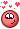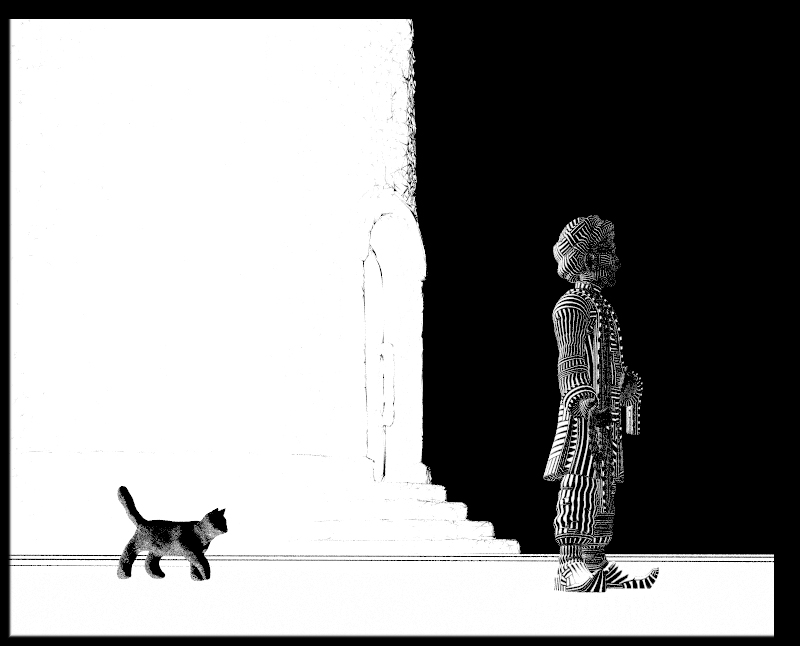## Recommended Posts

Here on the forum, you can find everythingjust to learn to combine /close.reading from points attribute Qfields FUN

```#define PI          3.1415926535897931
#pragma label       Theta       "Maximum Theta"
#pragma label       DATA        "Data Points"
#pragma label       kd          "Diffuse"
#pragma label       ks          "Specular"
#pragma label       bump        "Bump"

surface
qrotation_field
(   float   Theta   = 2.0,
kd      = 1,
ks      = 0.5,
bump    = 0.1;
{
float d, Np, theta, pFratt, factor, pspread;
vector _P, pP, axis, pVratt;
vector4 Q;
string group;

_P = ptransform("space:camera", "space:world", P);

theta = snoise(_P + 0.9, 1, 0.75, 1)*4.5;
axis = snoise(_P + 0.05, 1, 0.05, 1);
Q = quaternion(theta, axis);
_P = qrotate(Q, _P);

for(int g = 0; g < 2; g++){
group = concat("group", itoa(g));
int p[] = expandpointgroup(DATA, group);
Np = len(p);
factor = g + 1;
for (int i = 0; i < Np; i++){
pP = point(DATA, "P", p[i]);
axis = point(DATA, "N", p[i]);
pFratt = point(DATA, "fratt", p[i]);
pVratt = point(DATA, "vratt", p[i]);
d = distance2(pP, _P);
theta = 2*PI*(pFratt*2 - 1)*d*Theta/factor;
Q = quaternion(theta, axis);
_P = qrotate(Q, _P - pP) + pP;
}
}

vector outColor = snoise(_P*0.25, 1, 0.25, 1);

vector Nn = normalize(computenormal(P + min(outColor)*bump, N, Ng));

vector diff = diffuse(Nn)*outColor*dot(normalize(-I), N);

vector spec = specular(Nn, normalize(-I), fit01(min(outColor), 0.5, 0.1))*0.1;
spec += specular(N, normalize(-I), 0.05);

Cf = diff*kd + spec*ks;
}```

•1
##### Share on other sites

Interesting topic, thanks for sharing!

## Join the conversation

You can post now and register later. If you have an account, sign in now to post with your account.
Note: Your post will require moderator approval before it will be visible.×   Pasted as rich text.   Paste as plain text instead

Only 75 emoji are allowed.

×   Your previous content has been restored.   Clear editor

×   You cannot paste images directly. Upload or insert images from URL.

×
×